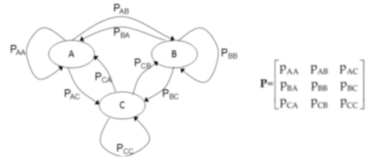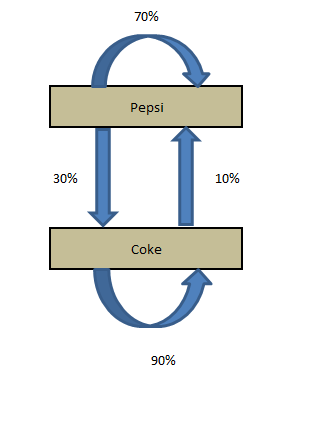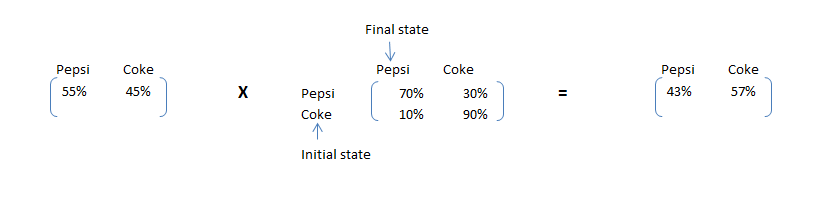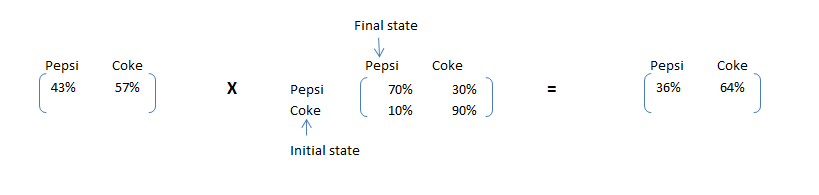×

Search anything:

# Markov Chain

#### Machine Learning (ML) markov chain probability statisticsGet this book -> Problems on Array: For Interviews and Competitive Programming

A Markov chain is a stochastic model describing a sequence of possible events in which the probability of each event depends only on the state attained in the previous event.The term "Markov chain" refers to the sequence of random variables such a process moves through, with the Markov property defining serial dependence only between adjacent periods (as in a "chain"). It can thus be used for describing systems that follow a chain of linked events, where what happens next depends only on the current state of the system.

# Indroduction

Markov chain is a simple concept which can explain most complicated real time processes.Speech recognition, Text identifiers, Path recognition and many other Artificial intelligence tools use this simple principle called Markov chain in some form. In this article we will illustrate how easy it is to understand this concept.Markov chain is based on a principle of “memorylessness”. In other words the next state of the process only depends on the previous state and not the sequence of states. This simple assumption makes the calculation of conditional probability easy and enables this algorithm to be applied in number of scenarios.

Coke and Pepsi are the only companies in country X. A soda company wants to tie up with one of these competitor. They hire a market research company to find which of the brand will have a higher market share after 1 month. Currently, Pepsi owns 55% and Coke owns 45% of market share. Following are the conclusions drawn out by the market research company:

P(P->P) : Probability of a customer staying with the brand Pepsi over a month = 0.7

P(P->C) : Probability of a customer switching from Pepsi to Coke over a month = 0.3

P(C->C) : Probability of a customer staying with the brand Coke over a month = 0.9

P(C->P) : Probability of a customer switching from Coke to Pepsi over a month = 0.1

We can clearly see customer tend to stick with Coke but Coke currently has a lower wallet share. Hence, we cannot be sure on the recommendation without making some transition calculations.

### Transition diagram

The four statements made by the research company can be structured in a simple transition diagram.The diagram simply shows the transitions and the current market share. Now, if we want to calculate the market share after a month, we need to do following calculations :

Market share (t+1) of Pepsi = Current market Share of Pepsi * P(P->P) + Current market Share of Coke * P(C->P)

Market share (t+1) of Coke = Current market Share of Coke * P(C->C) + Current market Share of Pepsi * P(P->C)

These calculations can be simply done by looking at the following matrix multiplication :

Current State X Transition Matrix = Final StateAs we can see clearly see that Pepsi, although has a higher market share now, will have a lower market share after one month. This simple calculation is called Markov chain. If the transition matrix does not change with time, we can predict the market share at any future time point. Let’s make the same calculation for 2 months later.# End Notes:

In this article we will restrict ourself to simple Markov chain. In real life problems we generally use Latent Markov model, which is a much evolved version of Markov chain. We will also talk about a simple application of Markov chain in the next article.#### Prashant Anand

Bachelor of Technology (2016 to 2020) in Electronics and Communications Engineering at Reva University, Bangalore | Intern at OpenGenus